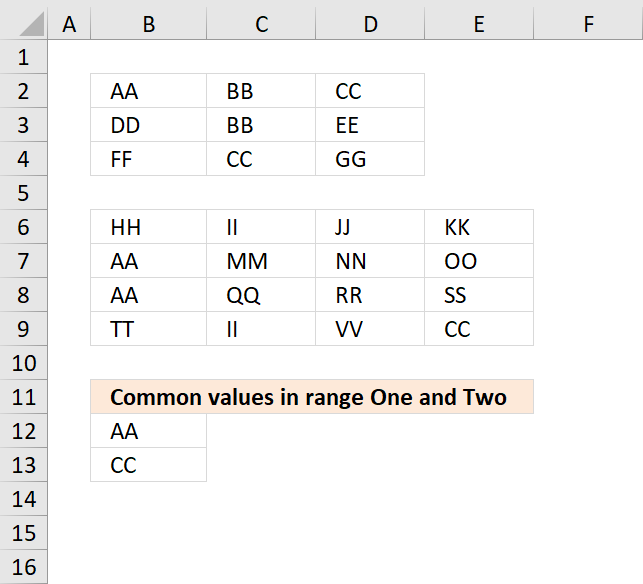Author: Oscar Cronquist Article last updated on December 19, 2018The image above shows an array formula in cell B12 that extracts values shared by cell range B2:D4 (One) and B6:E9 (Two). There is a much smaller formula if you only need to compare two columns: Extract shared values between two columns

Array formula in B12:

=TEXTJOIN("", TRUE, IF(MIN(IF(((COUNTIF(\$B\$6:\$E\$9, \$B\$2:\$D\$4)>0)+COUNTIF(B11:\$B\$11, \$B\$2:\$D\$4))=1, (ROW(\$B\$2:\$D\$4)+(1/(COLUMN(\$B\$2:\$D\$4)+1)))*1, ""))=(ROW(\$B\$2:\$D\$4)+(1/(COLUMN(\$B\$2:\$D\$4)+1)))*1, \$B\$2:\$D\$4, ""))

If your Excel version doesn't contain the TEXTJOIN function then use the following array formula.

Array formula in B12:

=INDEX(\$B\$2:\$D\$4, MIN(IF((COUNTIF(\$B\$6:\$E\$9, \$B\$2:\$D\$4)>0)+COUNTIF(B11:\$B\$11, \$B\$2:\$D\$4)=1, ROW(\$B\$2:\$D\$4)-MIN(ROW(\$B\$2:\$D\$4))+1, "")), MATCH(0, NOT(COUNTIF(\$B\$6:\$E\$9, INDEX(\$B\$2:\$D\$4, MIN(IF((COUNTIF(\$B\$6:\$E\$9, \$B\$2:\$D\$4)>0)+COUNTIF(B11:\$B\$11, \$B\$2:\$D\$4)=1, ROW(\$B\$2:\$D\$4)+1, ""))-MIN(ROW(\$B\$2:\$D\$4)), , 1)))+COUNTIF(B11:\$B\$11, INDEX(\$B\$2:\$D\$4, MIN(IF((COUNTIF(\$B\$6:\$E\$9, \$B\$2:\$D\$4)>0)+COUNTIF(B11:\$B\$11, \$B\$2:\$D\$4)=1, ROW(\$B\$2:\$D\$4)+1, ""))-MIN(ROW(\$B\$2:\$D\$4)), , 1)), 0))

To enter an array formula, type the formula in a cell then press and hold CTRL + SHIFT simultaneously, now press Enter once. Release all keys.

The formula bar now shows the formula with a beginning and ending curly bracket telling you that you entered the formula successfully. Don't enter the curly brackets yourself.

### Explaining formula in cell B12

#### Step 1 - Identify values shared by both cell ranges

The COUNTIF function counts values based on a condition or criteria, in this case, it compares values between the cell ranges.

COUNTIF(\$B\$6:\$E\$9, \$B\$2:\$D\$4)>0

becomes

COUNTIF({"HH", "II", "JJ", "KK";"AA", "MM", "NN", "OO";"AA", "QQ", "RR", "SS";"TT", "II", "VV", "CC"}, {"AA", "BB", "CC";"DD", "BB", "EE";"FF", "CC", "GG"})>0

becomes

{2,0,1;0,0,0;0,1,0}>0

and returns

{TRUE, FALSE, TRUE;FALSE, FALSE, FALSE;FALSE, TRUE, FALSE}

#### Step 2 - Prevent duplicates

The next COUNTIF function counts values based on a condition or criteria, in this case, we take into account previously displayed values in order to prevent duplicates from showing up in our list.

The first argument in the COUNTIF function B\$11:\$B11 expands as the cell is copied to cells below, this makes the formula aware of values above the current cell.

COUNTIF(\$B\$11:B11,\$B\$2:\$D\$4)=1

becomes

COUNTIF("Common values in range One and Two", {"AA", "BB", "CC";"DD", "BB", "EE";"FF", "CC", "GG"})=1

becomes

{0,0,0;0,0,0;0,0,0}=1

and returns

{FALSE, FALSE, FALSE;FALSE, FALSE, FALSE;FALSE, FALSE, FALSE}.

#### Step 3 - Add arrays

If at least one of the COUNTIF functions return TRUE then the array returns TRUE or the equivalent value 1.

(COUNTIF(\$B\$6:\$E\$9,\$B\$2:\$D\$4)>0)+COUNTIF(B11:\$B\$11,\$B\$2:\$D\$4))=1

becomes

({TRUE, FALSE, TRUE;FALSE, FALSE, FALSE;FALSE, TRUE, FALSE}+{FALSE, FALSE, FALSE;FALSE, FALSE, FALSE;FALSE, FALSE, FALSE})=1

becomes

{1,0,1;0,0,0;0,1,0}=1

and returns

{TRUE, FALSE, TRUE;FALSE, FALSE, FALSE;FALSE, TRUE, FALSE}.

#### Step 4 - Replace TRUE with coordinates

The following IF function returns the corresponding coordinate if boolean value is TRUE. FALSE returns "" (nothing).

IF(((COUNTIF(\$B\$6:\$E\$9,\$B\$2:\$D\$4)>0)+COUNTIF(B11:\$B\$11,\$B\$2:\$D\$4))=1,(ROW(\$B\$2:\$D\$4)+(1/(COLUMN(\$B\$2:\$D\$4)+1)))*1,"")

becomes

IF({TRUE, FALSE, TRUE;FALSE, FALSE, FALSE;FALSE, TRUE, FALSE},(ROW(\$B\$2:\$D\$4)+(1/(COLUMN(\$B\$2:\$D\$4)+1)))*1,"")

The ROW and COLUMN functions return the row(s) and column(s) of a cell range. Adding these two numbers creates a unique number for each cell in the cell range.

IF({TRUE, FALSE, TRUE;FALSE, FALSE, FALSE;FALSE, TRUE, FALSE},(ROW(\$B\$2:\$D\$4)+(1/(COLUMN(\$B\$2:\$D\$4)+1)))*1,"")

becomes

IF({TRUE, FALSE, TRUE;FALSE, FALSE, FALSE;FALSE, TRUE, FALSE},{2.33333333333333, 2.25, 2.2;3.33333333333333, 3.25, 3.2;4.33333333333333, 4.25, 4.2},"")

and returns

{2.33333333333333,"",2.2;"","","";"",4.25,""}

#### Step 5 - Get the smallest value in array

The MIN function returns the smallest number in array ignoring blanks and text values.

MIN(IF(((COUNTIF(\$B\$6:\$E\$9,\$B\$2:\$D\$4)>0)+COUNTIF(B11:\$B\$11,\$B\$2:\$D\$4))=1,(ROW(\$B\$2:\$D\$4)+(1/(COLUMN(\$B\$2:\$D\$4)+1)))*1,""))

becomes

MIN({2.33333333333333,"",2.2;"","","";"",4.25,""})

and returns 2.2.

#### Step 6 - Identify value in cell range

IF(MIN(IF(((COUNTIF(\$B\$6:\$E\$9, \$B\$2:\$D\$4)>0)+COUNTIF(B11:\$B\$11, \$B\$2:\$D\$4))=1, (ROW(\$B\$2:\$D\$4)+(1/(COLUMN(\$B\$2:\$D\$4)+1)))*1, ""))=(ROW(\$B\$2:\$D\$4)+(1/(COLUMN(\$B\$2:\$D\$4)+1)))*1, \$B\$2:\$D\$4, "")

becomes

IF(2.2=(ROW(\$B\$2:\$D\$4)+(1/(COLUMN(\$B\$2:\$D\$4)+1)))*1,\$B\$2:\$D\$4,"")

becomes

IF(2.2={2.33333333333333, 2.25, 2.2; 3.33333333333333, 3.25, 3.2; 4.33333333333333, 4.25, 4.2},\$B\$2:\$D\$4,"")

becomes

IF({FALSE,FALSE, TRUE;FALSE, FALSE,FALSE; FALSE,FALSE, FALSE},\$B\$2:\$D\$4,"")

and returns

{"","","CC";"","","";"","",""}.

#### Step 7 - Join text strings in array

The TEXTJOIN function returns values concatenated ignoring blanks in array.

TEXTJOIN("", TRUE, IF(MIN(IF(((COUNTIF(\$B\$6:\$E\$9, \$B\$2:\$D\$4)>0)+COUNTIF(B11:\$B\$11, \$B\$2:\$D\$4))=1, (ROW(\$B\$2:\$D\$4)+(1/(COLUMN(\$B\$2:\$D\$4)+1)))*1, ""))=(ROW(\$B\$2:\$D\$4)+(1/(COLUMN(\$B\$2:\$D\$4)+1)))*1, \$B\$2:\$D\$4, ""))

becomes

TEXTJOIN("", TRUE, {"","","CC";"","","";"","",""})

and returns "CC" in cell B12.